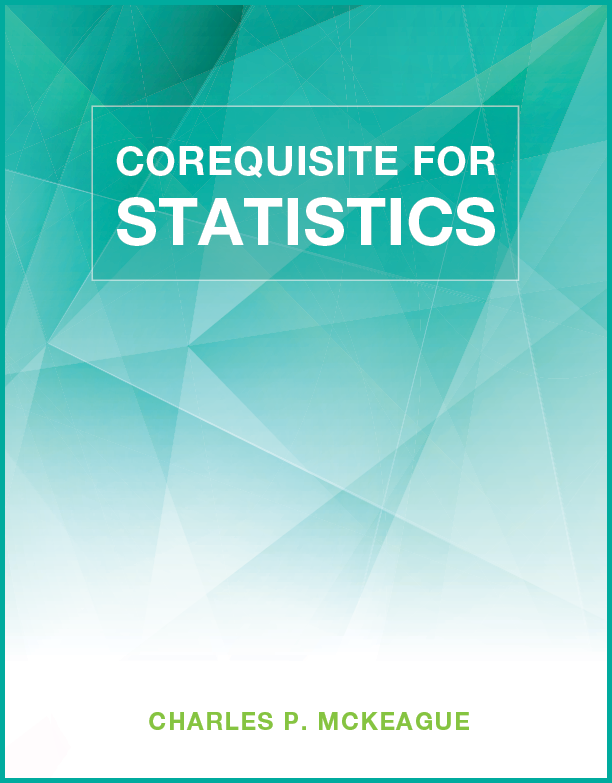#### Videos for:Corequisite for Statistics
Definitions, Order of Operations, Simplifying Expressions, and Sets

Select an Instructor to Play Video

Example 1

Write each of the following in symbols:

The sum of $$x$$ and $$5$$ is less than $$2$$.
The product of $$3$$ and $$x$$ is $$21$$.
The quotient of $$y$$ and $$6$$ is $$4$$.
Twice the difference of $$b$$ and $$7$$ is greater than $$5$$.
The difference of twice $$b$$ and $$7$$ is greater than $$5$$.

Example 14

If $$A=\{\text{all college students in the United States}\}$$ and $$B=\{\text{all college students in Boston}\}$$, then which of the following is true?

1. $$A\subset B$$

2. $$B\subset A$$

Mini Lecture
Simplify.

1. $$4\cdot 2^2+5\cdot 2^3$$

2. $$40-10\div 5+1$$

3. $$3\left[2+4(5+2\cdot 3)\right]$$

4. Translate into symbols: The sum of $$x$$ and $$y$$ is less than the difference of $$x$$ and $$y$$.Innovative products, peer tutors, fair prices, and unlimited access
www.xyztextbooks.com | [email protected]